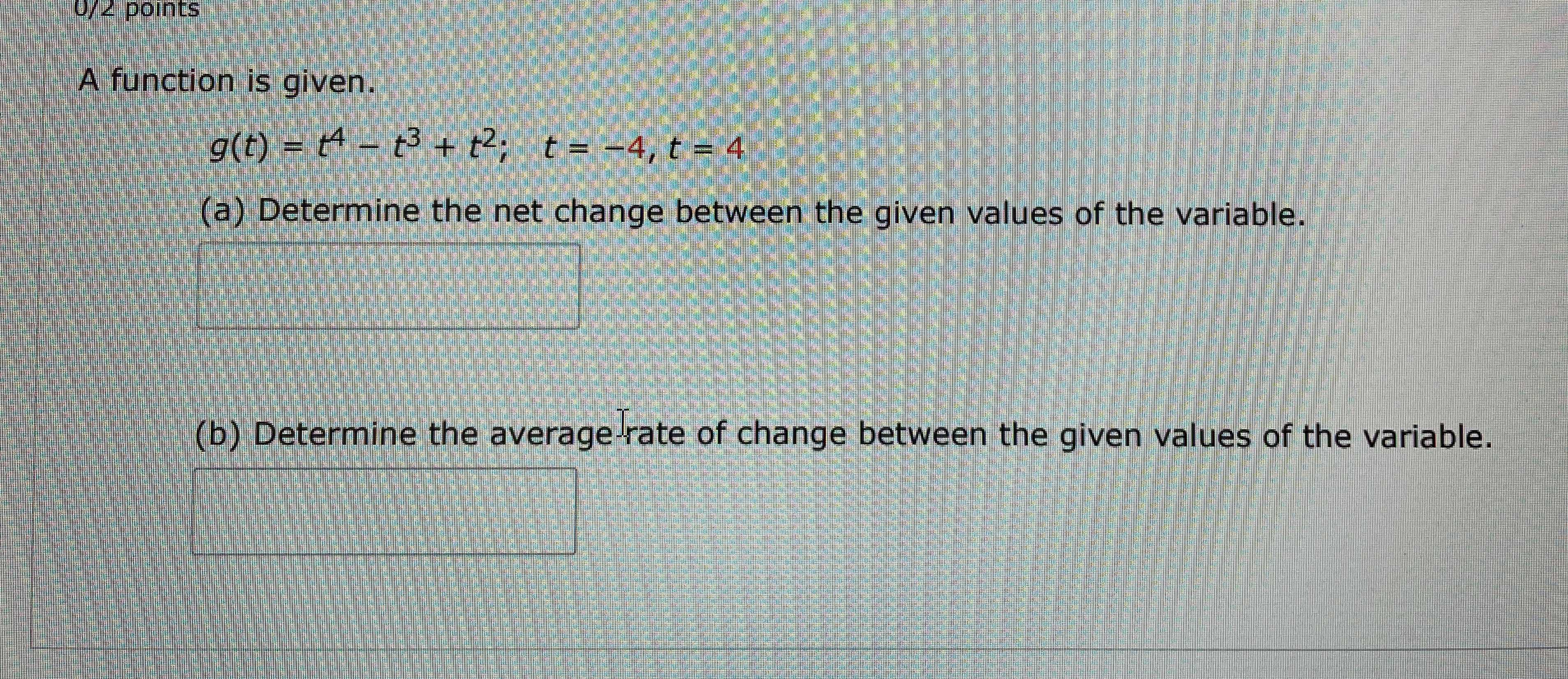### ¿Todavía tienes preguntas de matemáticas?

Pregunte a nuestros tutores expertos
Algebra
PreguntaA function is given.

$$g ( t ) = t ^ { 4 } - t ^ { 3 } + t ^ { 2 } ; t = - 4 , t = 4$$

(a) Determine the net change between the given values of the variable.

(b) Determine the average Irate of change between the given values of the variable.